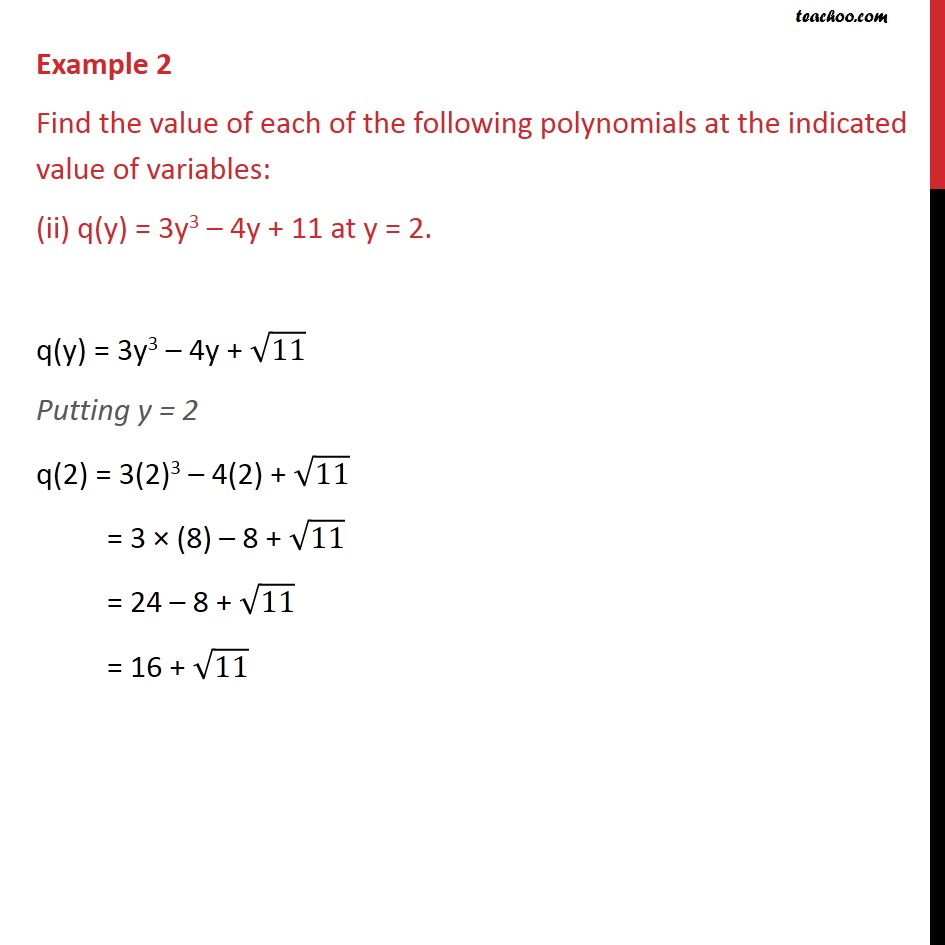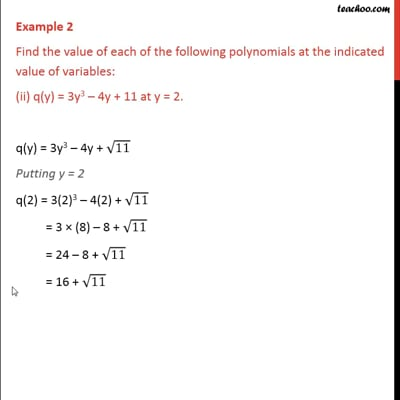Examples

Chapter 2 Class 9 Polynomials
Serial order wiseThis video is only available for Teachoo black users

Maths Crash Course - Live lectures + all videos + Real time Doubt solving!

### Transcript

Example 2 Find the value of each of the following polynomials at the indicated value of variables: (ii) q(y) = 3y3 – 4y + 11 at y = 2. q(y) = 3y3 – 4y + √11 Putting y = 2 q(2) = 3(2)3 – 4(2) + √11 = 3 × (8) – 8 + √11 = 24 – 8 + √11 = 16 + √11